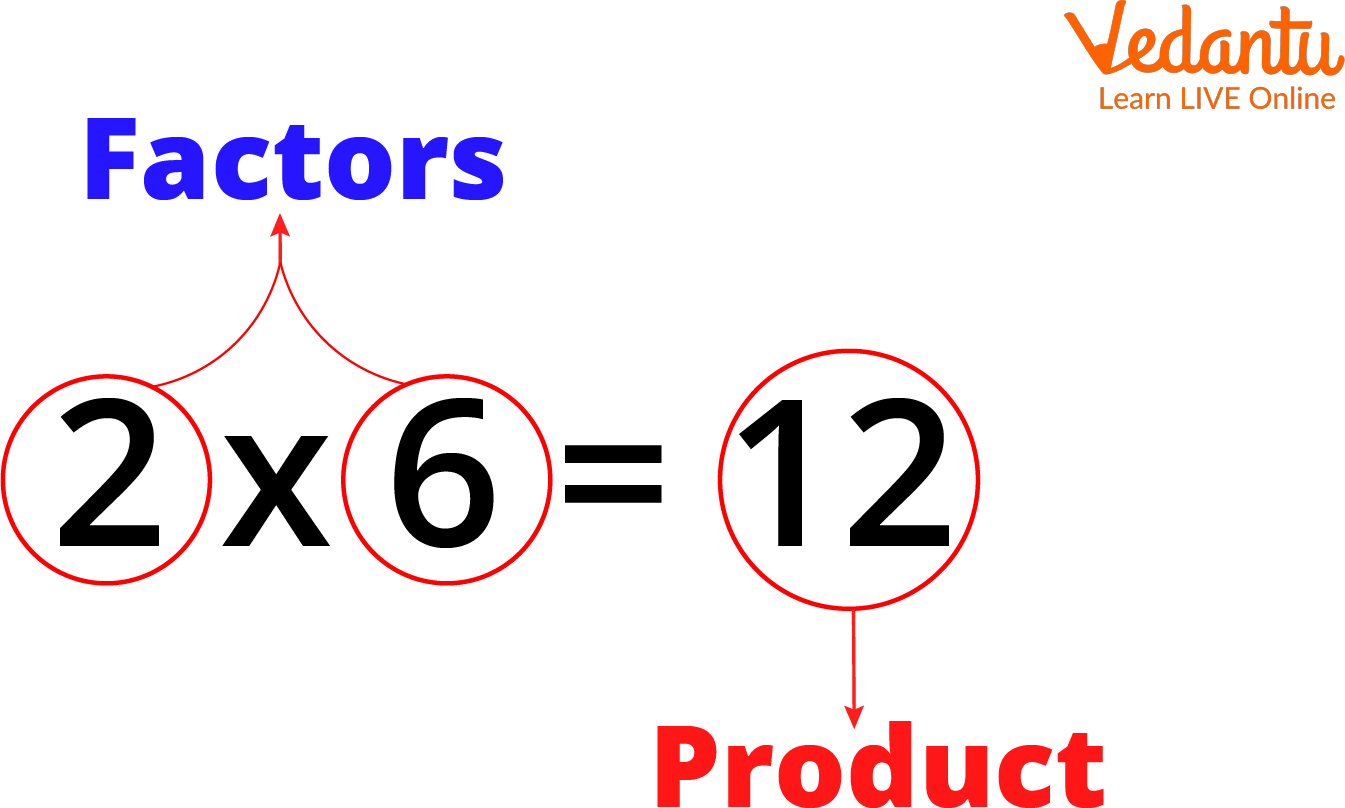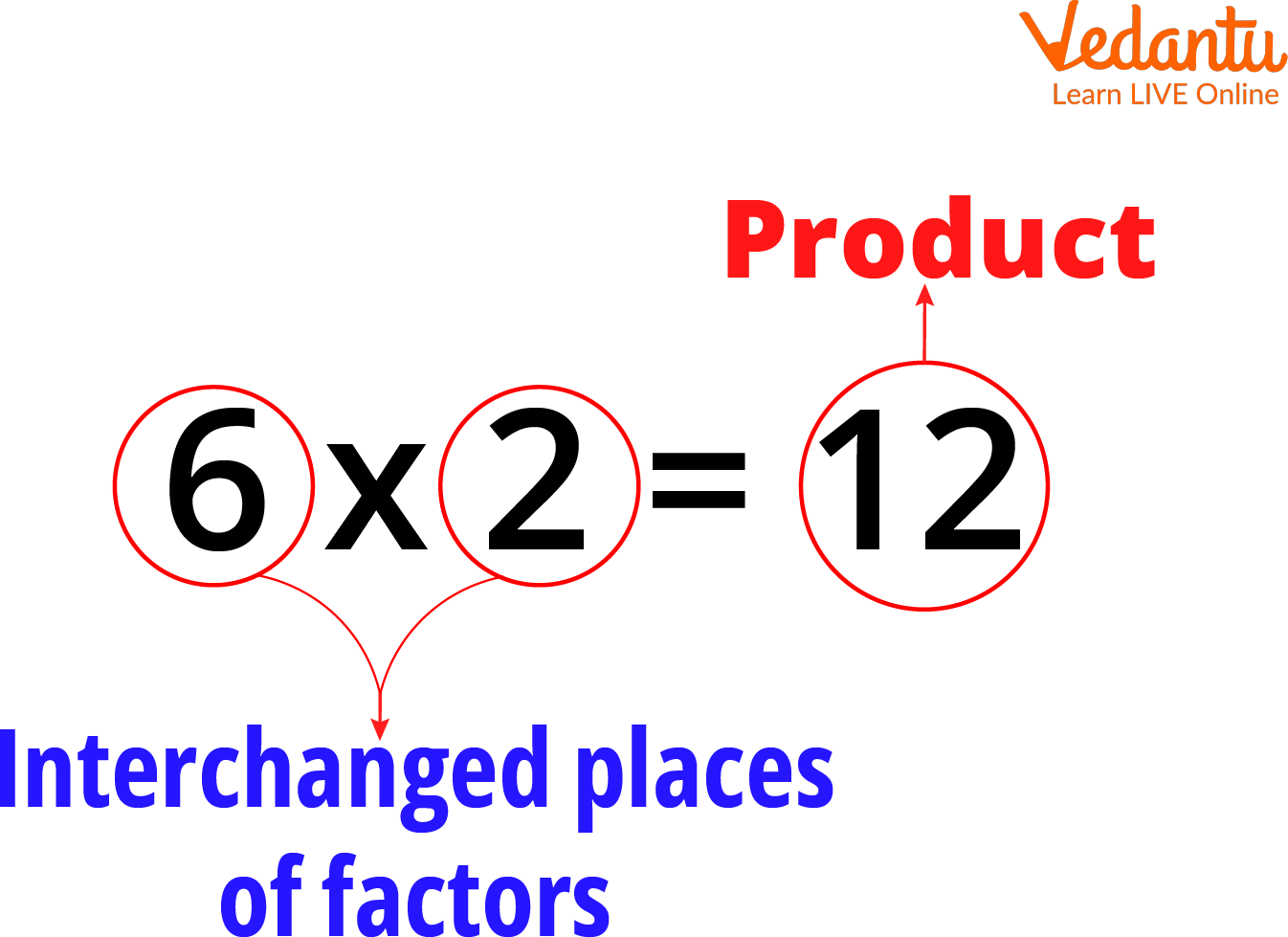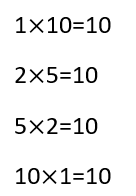Courses
Courses for Kids
Free study material
Free LIVE classes
More

# Factors by Multiplication Method## Introduction to Factors by Multiplication

Factors can be defined as the numbers that divide a number without leaving any remainder. These numbers are usually whole numbers, which, when multiplied with other whole numbers, give products. We can find the factors by using methods like factorization, multiplication tables, and division. The first and simplest method for finding factors is by using the multiplication method. Today you will learn to find factors by the multiplication method.

## What are Factors and Multiplication Facts?

As said above, when two whole numbers are multiplied to give us a product, the whole numbers are called the factors. For example, have a look at the image below.Factors and Their Product

In the image above, you can see that 2 and 6 are multiplied together to give 12 as a product, so we can write that 2 and 6 are the factors of 12. If you know about multiplication, you must already know that 2 is a multiplicand, 6 is the multiplier, and 12 is the product. If you need to learn about multiplicands and multipliers, observe that 2 in the above picture is multiplied by 6 and gives 12. So, 2 is the multiplicand, 6 is the multiplier, and 12 is the product. Therefore, a multiple or a multiplication factor is a product of multiplication factors.

And according to the definition of factor, both multiplicand and multiplier are factors. The multiplicand and multiplier can also change places, as shown below.Changed Places of Multiplicand and Multiplier

So, this is what a multiplication factor is. On the other hand, the product is known as multiple or multiplication facts. This is how you can identify factors and multiplication facts.

## How to Find Factors and Multiples by Using Multiplication Facts?

Have a look at the chart below. You can see that all the multiplication results are 10, and these are the multiplication facts. You can see that 1, 2, 5, and 10 are multiplied to get the multiplication fact of 10. Hence, 1, 2, 5, and 10 are the factors of 10.Image: Factors and multiples chart,

Therefore, to calculate the factors using multiplication facts, you have to write the multiplication facts and their multiples. First, you must start with one because one is a factor in every number. Then check and proceed with every number one by one, as done above. This is how you can use the multiplication method to find factors with the help of multiplication facts.

## Examples of Factors and Multiplication Facts

Let us see some examples where you can use multiplication facts to find factors.

(i) Determine the factors of 15

$1 \times 15 = 15$.

You can see that 1 and 15 are factors of 15.

We can also write that

$3 \times 5 = 15$

Hence, the above expression was put in place of 15 on the left side.

Thus, the factors of 15 are 1, 15, 3, and 5.

(ii) Find factors of 24.

$1 \times 24 = 24$

You can expand the above expression by checking the divisibility one by one,

$2 \times 12 = 24$

One more expression that can be written is,

$3 \times 8 = 24$

Therefore, the factors of 24 are 1, 2, 3, 8, 12, and 24.

## Conclusion

You have learnt that we can find factors using division and multiplication. You have learnt about factors, multiplication facts, multiplicands, multipliers, and multiples. The product of multiplication is called multiple, and multiplicands and multipliers are called factors. Multiplication facts determine the factors if you use the multiplication method.

Last updated date: 25th May 2023
Total views: 42k
Views today: 0.41k

## FAQs on Factors by Multiplication Method

1. Can we write all the factors in one line together?

Yes! The factors can be written all in one line by further expanding the greater multiplier on the left side. For example, if there is 12 on the left side of the expression, 12 can be expanded to 3 and 4. The 4 can be further expanded to 2 and 2. Hence, all the numbers at the end will be the factors.

2. What minimum number of factors can a multiplication fact have?

The minimum number of factors a multiplication fact can have is two. The two factors are 1 and the number itself. For example, the number 13 has two factors, 1 and 13 itself, because it is not divisible by any other number. Another example is 14, which has 1, 2, 7, and 14 as factors, resulting in 4 factors. Therefore, the minimum number of factors of any number is 2.

3. What is the maximum number of factors that a multiplication fact can have?

The maximum number of factors a multiplication fact can have is infinity. A number can have any number of factors, but the number of factors cannot be less than 2.